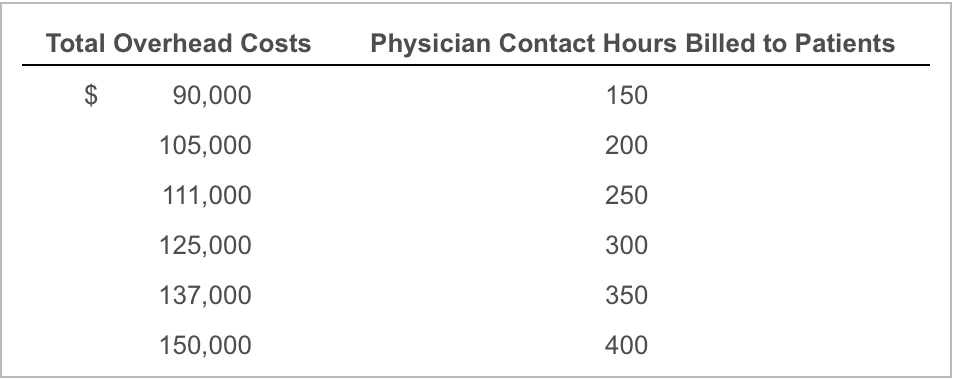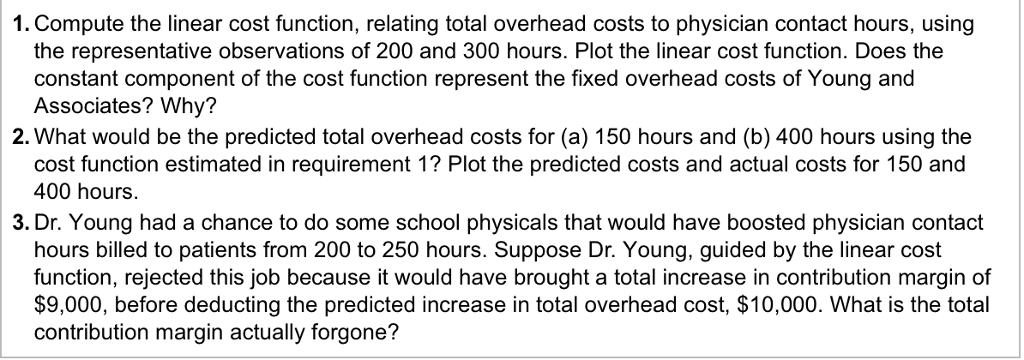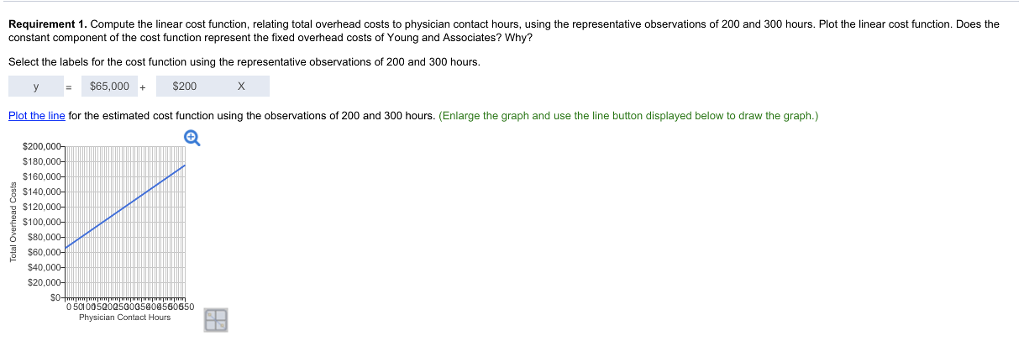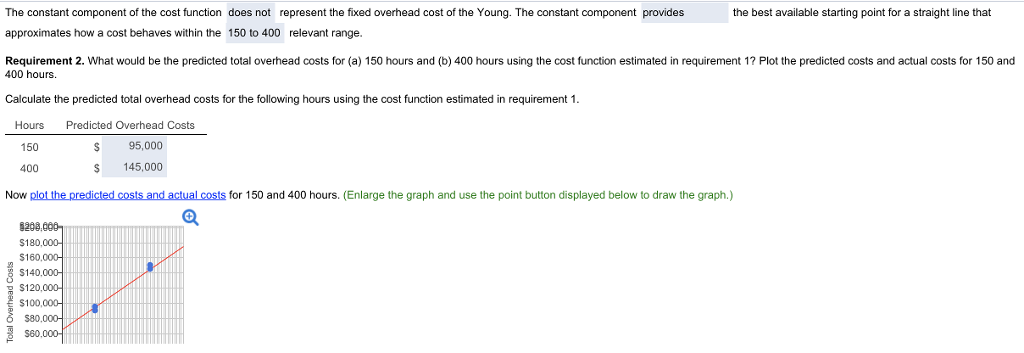# Question & Answer: Dr. Young, of Young and Associates, LLP, is examining how overhead costs behave as a function of monthly physician contact hour…..

How to calculate the actual cost at 150 and 400 hours?Don't use plagiarized sources. Get Your Custom Essay on
Question & Answer: Dr. Young, of Young and Associates, LLP, is examining how overhead costs behave as a function of monthly physician contact hour…..
GET AN ESSAY WRITTEN FOR YOU FROM AS LOW AS \$13/PAGEDr. Young, of Young and Associates, LLP, is examining how overhead costs behave as a function of monthly physician contact hours billed to patients. The historical data are as follows 囲(Click the icon to view the data.) Read the requirements.

First we find the cost function using the 200 and 300 physical contact hrs

Let the linear equation is y=a+bx

x= no of physical hrs

105000=a+200b

125000=a+300b

Solving we get b=200 and a=65000

The linear function is y=65000+200x

For 150 hrs the cost is=65000+(150*200)=95000

For 400 hrs the cost is =65000+(400*200)=145,000